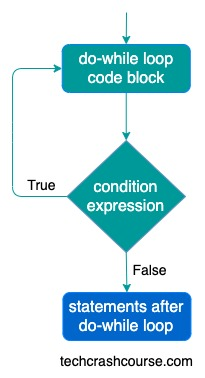# Java Do While Loop

Do..While loop in java is similar to while loop except do-while loop evaluates its condition expression after the execution of loop code block. Do..While loop in java is used to execute a block of code multiple times.

Do while loop ensures that that code block inside do-while body will execute atleast once.

As the condition expression check is after do-while loop code block, it first execute it's code block and then decide whether to iterate again or not.

Syntax of do-while Loop in Java
```do {
// do while loop code block
statement(s);
} while(condition);
```

## Control flow of do-while loop

condition expression is a boolean expression which gets evaluated every time after loop code block. Here is the description of do..while loop control flow.

• First of all, do-while loop code block gets executed.
• do-while loop evaluates condition expression inside the parenthesis.
• If condition expression evaluates to true, then do-while loop code block gets executed.
• condition expression is evaluated again.
• This execution process continues until the condition expression evaluates false.
• Whenever condition expression evaluates to false, do-while loop terminates and control goes to the next statement after the do-while loop.

Here is the flowchart of do-while loop execution### Java Do-While Loop Example Program

```public class DoWhileLoop {
public static void main(String[] args) {
int count = 0;
do {
System.out.println(count);
count++;
} while(count < 5);
}
}
```
Output
```0
1
2
3
4
```

In above program, do-while loop executes code block 5 times, till the time the value of count variable is less than 5. With each iteration the value of count variable is incremented. As soon as value of count becomes 5 do-while loop terminates.

Here are the execution steps for each iteration of do-while loop in above program.

Iteration Count Variable Do-While Code Block Condition(count < 5)
1 count = 0 Print count(0).
count is incremented to 1
true
2 count = 1 Print count(1).
count is incremented to 2
true
3 count = 2 Print count(2).
count is incremented to 3
true
4 count = 3 Print count(3).
count is incremented to 4
true
5 count = 4 Print count(4).
count is incremented to 5
false
• Unlike for loop, there is no initialization and update statements.

• The condition in do-while loop is a boolean expression.

• We can also use infinite do while loops like while(1), which will never terminate. You should add terminating condition using break statement in order to terminate infinite do-while loop.
For Example:
do {
if(....){
break;
}
} while(1);

• Opening and Closing braces are not required for single statement inside do while loop code block.
For Example:
do
statement;
while(condition);